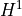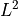# sfepy.discrete.projections module¶

Construct projections between FE spaces.

sfepy.discrete.projections.create_mass_matrix(field)[source]

Create scalar mass matrix corresponding to the given field.

Returns:
mtxcsr_matrix

The mass matrix in CSR format.

sfepy.discrete.projections.make_h1_projection_data(target, eval_data)[source]

Project scalar data given by a material-like eval_data() function to a scalar target field variable using thedot product.

sfepy.discrete.projections.make_l2_projection(target, source, ls=None, nls_options=None)[source]

Project a scalar source field variable to a scalar target field variable using thedot product.

sfepy.discrete.projections.make_l2_projection_data(target, eval_data, order=None, ls=None, nls_options=None)[source]

Project scalar data to a scalar target field variable using thedot product.

Parameters:
targetFieldVariable instance

The target variable.

eval_datacallable or array

Either a material-like function eval_data(), or an array of values in quadrature points that has to be reshapable to the shape required by order.

orderint, optional

The quadrature order. If not given, it is set to 2 * target.field.approx_order.

sfepy.discrete.projections.project_by_component(tensor, tensor_qp, component, order, ls=None, nls_options=None)[source]

Wrapper around make_l2_projection_data() for non-scalar fields.

sfepy.discrete.projections.project_to_facets(region, fun, dpn, field)[source]

Project a function fun to the field in facets of the given region.